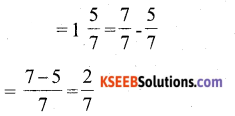# KSEEB Solutions for Class 6 Maths Chapter 7 Fractions Ex 7.5

Students can Download Chapter 7 Fractions Ex 7.5 Questions and Answers, Notes Pdf, KSEEB Solutions for Class 6 Maths helps you to revise the complete Karnataka State Board Syllabus and score more marks in your examinations.

## Karnataka State Syllabus Class 6 Maths Chapter 7 Fractions Ex 7.5

Question 1.
Write these fractions appropriately as additions or subtractions:-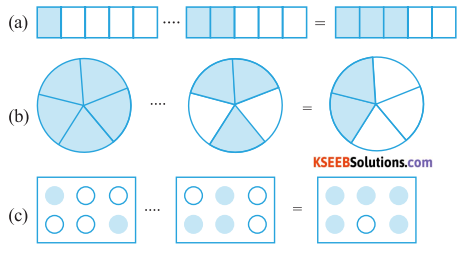Solution: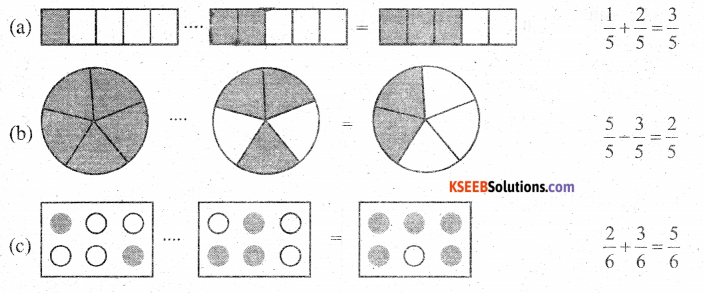Question 2.
Solve: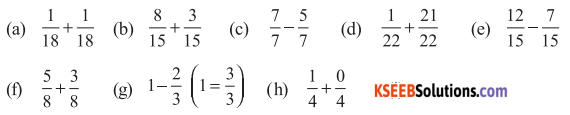Solution: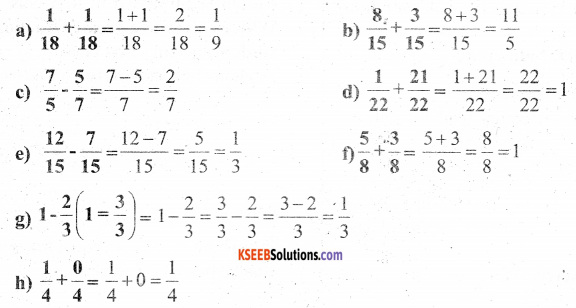Question 3.
Shubham Painted $$\frac{2}{3}$$ of the wall space in his room. His sister Madhavi helped and painted $$\frac{1}{3}$$ of the wall space. How much did they paint together?
Solution:
Space painted by Shubhan = $$\frac{2}{3}$$ of the room
Space painted by Madhavi = $$\frac{1}{3}$$ of the room
Hence, together they painted = $$\left(\frac{2}{3}+\frac{1}{3}\right)$$ of the room
= 1 = the complete wall.

Question 4.
Fill in the missing fraction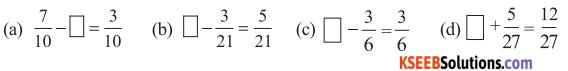Solution: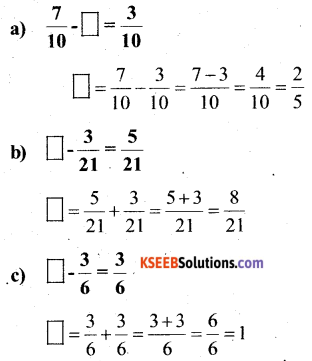d)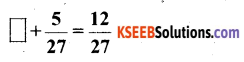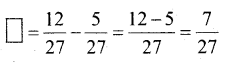Question 5.
Javed was given $$\frac{5}{7}$$ of a basket of oranges. What fraction of oranges was left in the basket?
Solution:
Fraction given to Javed = $$\frac{5}{7}$$